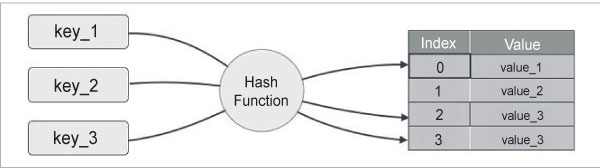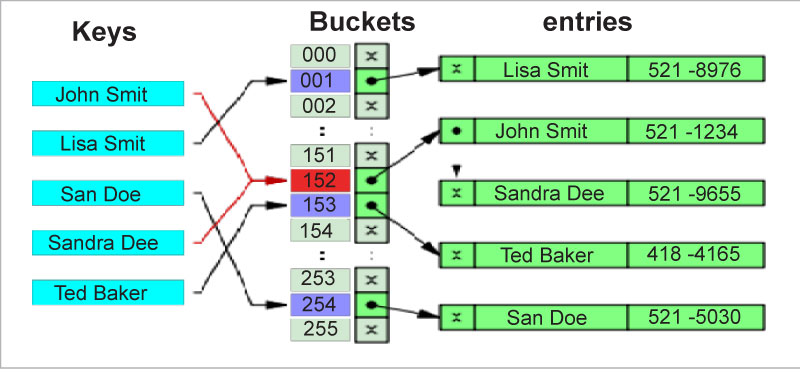HomeAudienceDevelopersWorking with Hash in C

# Working with Hash in C

Hash is the most commonly used data structure in everyday programming. If you are a Python developer, every time you use dict or set, hash is used internally. In Perl, you call it hash using {} and in Java it’s called HashSet or HashMap.

There are different ways to save data — for example, in a variable, as arrays, as structures, and so on. Hash is yet another way to save data. So if we have a key-value pair, we can use hash for quick save and retrieval.

There are three perquisites for hashing, as given below:

• Key
• Value
• Hash function

Key: Unique data to store the value
Value: Data which needs to be associated with the key
Hash function: Function used to compute the hash code from the given key. The given hash code is the index to save the value. For each key, the hash function is expected to return a unique hash code (index).Figure 1: Each key is passed through a hash function and index values are recorded based on the outcome [https://www.tutorialspoint.com/data_structures_algorithms/hash_data_structure.htm]Hash Collision
Hash Collision is a flaw of the hash function which generates the same hash code for two different keys. As an example, we have two keys k1 and k2. If h(k1) and h(k2) generate the same hash code, how can data be stored and retrieved? A good hash function is one that minimises the collisions and spreads the records uniformly throughout the table.
There are two basic techniques to resolve this problem, rehashing and chaining.

Rehashing: Rehashing is the easiest method as it places the record in the next available position of the array. This technique is also called linear probing. If array location h(key) is already occupied by a record, then a general rehash (rh) function rh(h(key)) is called. If this is also occupied, then rh(rh(h(key))) is called. However, it is possible for the rehash function to be called in a loop indefinitely, even if there are many empty functions.

Chaining: The other method of resolving the hash clashes is called chaining. It involves keeping a distinct linked list of all the records whose keys hash to the particular value.

Figure 2 gives a nice representation of a chained hash table.Figure 2: A small phone book as hash table using chaining mechanism[https://en.wikipedia.org/wiki/Hash_table]Algorithm
Pseudo code:

• Create an array of the structure
• Define a hash function to accept a key as the input and return the index
 Note: There are several ways to define a hash function; we have chosen a basic one.

Search function:

• Get the index by calling the hash function passing the key
• Validate if the index exists
• If it exists, loop through the index
• Match the given key looping through the linked list structure pointed to the index, to check if the key exists
• If found, return the structure address pointer
• Else return NULL

Insert function:

• Call the Search function passing the key to determine whether the given key is already present
• If it is present, override with the new value
• Else create a new entry
• Return NULL if there is no memory for the new entry

Source code:

```/* pointer array size */
#define HASHSIZE 101

/* structure to save the key and value */
struct nlist {
struct nlist *next; /* next entry in chain */
char *key; /* defined key */
char *value; /* replacement text which holds value */
}

/* pointer table to save list of structures */
static struct nlist *hashtab[HASHSIZE]

/* hash: form hash value for string s which is key */
unsigned hash(char *s) {
/* unsigned value ensures non negative */
unsigned hashval;

/* adds each character’s value of the string to form scrambled combination of the previous ones */
for (hashval =0; *s != ‘\0’; s++) {
hashval = *s + 31 * hashval;
}

/* modulo ensures the index is within the array size */
return hashval % HASHSIZE
}

/* lookup: look for s in the hash table */
struct nlist *lookup(char *s) {
struct nlist *np;

for (np = hashtab[hash[(s)]; np != NULL; np = np->next) {
if(strcmp(s, np->key) == 0) {
return np;
}
}

/* key not found in hash table */
return NULL;
}

/* insert: add the key and value to the hash table */
struct nlist *insert(char *key, char *value) {
struct nlist *np;
unsigned hashval;

/* call the lookup function to validate if already key exists */
/* if not exists allocate the memory for the new structure */
if ((np = lookup(key)) == NULL) {
np = (struct nlist *) malloc(sizeof*np));

/* if unable to allocate memory for the structure */
/* or unable to copy key in the struct key */
/* return NULL as it no more memory could be allocated */
if (np == NULL || (np->key = strdup(key)) == NULL) {
return NULL;
}

/* get the index for the key, by calling the hash function */
hashval = hash(key);

/* point the newly created structure’s next to the existing index value’s struct */
np->next = hashtab[hashval];

/* point the current pointer to the head of the index */
/* so the newly created pointer will be pointed as first */
hashtab[hashval] = np;
} else {
/* if already the key is available, free up its value so we can override below */
free((void *) np->value);
}

/* save the value to the key’s structure */
if((np->value = strdup(value)) == NULL) {
return NULL;
}
}```
 Note: The chaining mechanism is used above to avoid hash collisions.

Applications

Now that we have discussed hash and its implementation, let’s view its common applications. In cryptography, hash functions are used to produce the hashed output from the input, which is impossible to reverse. Password verification is another important use of cryptographic hash functions. When a password is entered by the client, it is hashed and sent to the server. As the server already knows the hash of the password, it can simply compare it. If there is a middle man attack, the password is still unknown to the hacker. The Rabin Karp string search algorithm uses hashing to find a set of patterns in the string. Its real world application is to detect plagiarism.